Ask your WordPress questions! Pay money and get answers fast!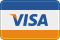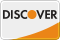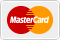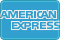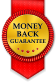# Gravity Forms Pre Populate Checkbox Select WordPress

I have a checkbox list (several of them) that have the values filled with the IDs from several different categories. I'm try to display them and if the value is in an array of the terms from a post (ID past in a query string) then it is selected. I'm having some trouble with the Array formatting.

Here is where I am:

```add_filter('gform_pre_render_2', 'GF_prepopulate_cat'); function GF_prepopulate_cat(\$form){ foreach(\$form["fields"] as &\$field){ if(\$field["id"] == 79 OR \$field["id"] == 80 OR \$field["id"] == 81 OR \$field["id"] == 82 OR \$field["id"] == 83 OR \$field["id"] == 84){ \$field['choices'] = array(); foreach ( \$field['choices'] as \$key => \$value){ if (isset(\$_GET["gform_post_id"])){ \$val = \$key->value; \$name = \$key->label; \$ID = \$_GET["gform_post_id"]; \$term_list = wp_get_post_terms(\$ID, 'portfolio-item-category', array("fields" => "ids")); if (in_array(\$val, \$term_list)) { \$select = true; } else { \$select = false; } \$field['choices'][] = array( 'text' => \$name, 'value' => \$val, 'isSelected' => \$select ); } } } } return \$form; }```

I believe it is all okay, except for the foreach setup

2013-07-04

first of all in the function

wp_get_post_terms(\$ID, 'portfolio-item-category', array("fields" => "ids"));

\$ID should be post id

\$ID = \$post_id;

and here is the modified code , hope it will work .
``` <?php add_filter('gform_pre_render_2', 'GF_prepopulate_cat'); function GF_prepopulate_cat(\$form){ foreach(\$form["fields"] as &\$field){ if(\$field["id"] == 79 OR \$field["id"] == 80 OR \$field["id"] == 81 OR \$field["id"] == 82 OR \$field["id"] == 83 OR \$field["id"] == 84){ \$field['choices'] = array(); foreach ( \$field['choices'] as \$key => \$value){ if (isset(\$_GET["gform_post_id"])){ \$val = \$key->value; \$name = \$key->label; \$term_list = wp_get_post_terms(\$ID, 'portfolio-item-category', array("fields" => "ids")); \$term_id = array(); if( !empty( \$term_list ) ){ foreach( \$term_list as \$tl ) { \$term_id[] = \$tl->term_id; } if (in_array(\$val, \$term_id)) { \$select = true; } else { \$select = false; } } \$field['choices'][] = array( 'text' => \$name, 'value' => \$val, 'isSelected' => \$select ); } } } } return \$form; } ?> ```

Sorry when I posted an edit update I copy and pasted over a line. \$ID is supposed to be this:

` \$ID = \$_GET["gform_post_id"];`

have you tried my solution , it worked?

It did not unfortunately

2013-07-04

``` ... \$choices = \$field['choices']; \$field['choices'] = array(); foreach ( \$choices as \$key => \$value){ if (isset(\$_GET["gform_post_id"])){ \$val = \$key->value; \$name = \$key->label; \$ID = \$_GET["gform_post_id"]; \$term_list = wp_get_post_terms(\$ID, 'portfolio-item-category', array("fields" => "ids")); if (in_array(\$val, \$term_list)) { \$select = true; } else { \$select = false; } \$field['choices'][] = array( 'text' => \$name, 'value' => \$val, 'isSelected' => \$select ); } } ... ```
```\$field['choices'] = array(); foreach ( \$field['choices'] as \$key => \$value){ ```